# How to Calculate Time in Excel?

• Last Updated : 29 Jun, 2021

In this article, we will look into methods for calculating time and time variance in Excel. In Excel, time is stored as numbers where day and part of the day are represented by the whole numbers and decimal numbers respectively. It is also important to note that the whole number 1 represents 01 Jan 1900 which is also the starting point from which Excel evaluates dates.

## Calculating Time Difference in Excel:

Here we will discuss a couple of methods used to calculate time difference in Excel.

Attention reader! Don’t stop learning now. If you are an Excel beginner (or an intermediate) and want to learn Excel, Geeksforgeeks brings the perfect course for you to start, Diving Into Excel

### 1. Simple Subtraction of Calculate Time Difference in Excel:

Time is stored as a number in Excel, to find the difference between 2 time values, one can easily subtract the start time from the end time.

Here, is the simple formula

`END TIME - START TIME`

In the below fig. we can see that

`=B2-A2 `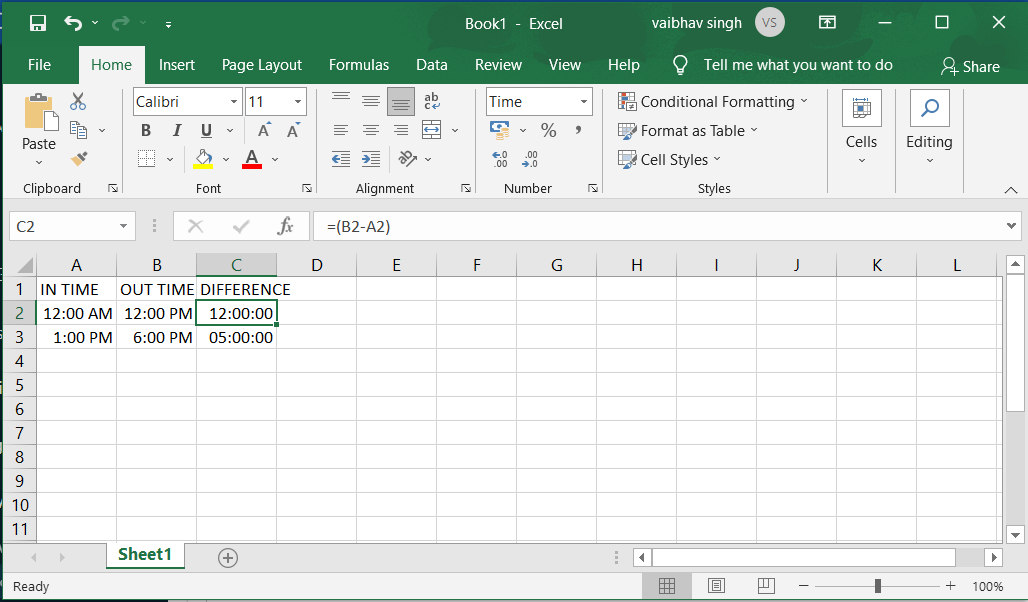There is a possibility that your results are shown in the time format (instead of decimals or in hours/minutes values).

### 2. Calculate the Time Difference in Hours, Minutes, or Seconds

1. Calculating Time Difference in Hours :

Below is the formula that will give you the time difference in hours:

`=(B2-A2)*24`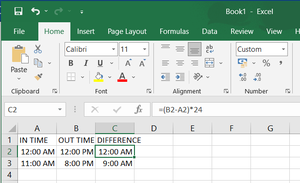2. Calculating Time Difference in Minutes:

Below is the formula that will give you the time difference in min:

`=(B2-A2)*60*24`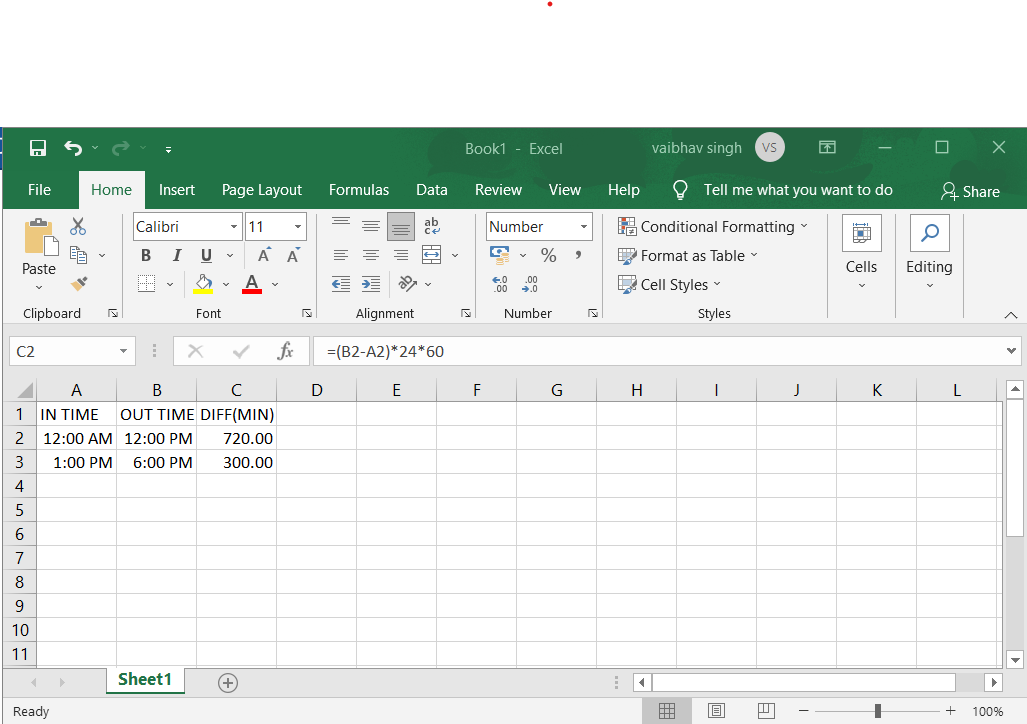3. Calculating Time Difference in Seconds:

Calculate the time difference in seconds, you need to multiply the resulting value by the total number of seconds in a day (which is or 24*60*60 or 86400). Below is the formula that will do that:

`=(B2-A2)*24*60*60`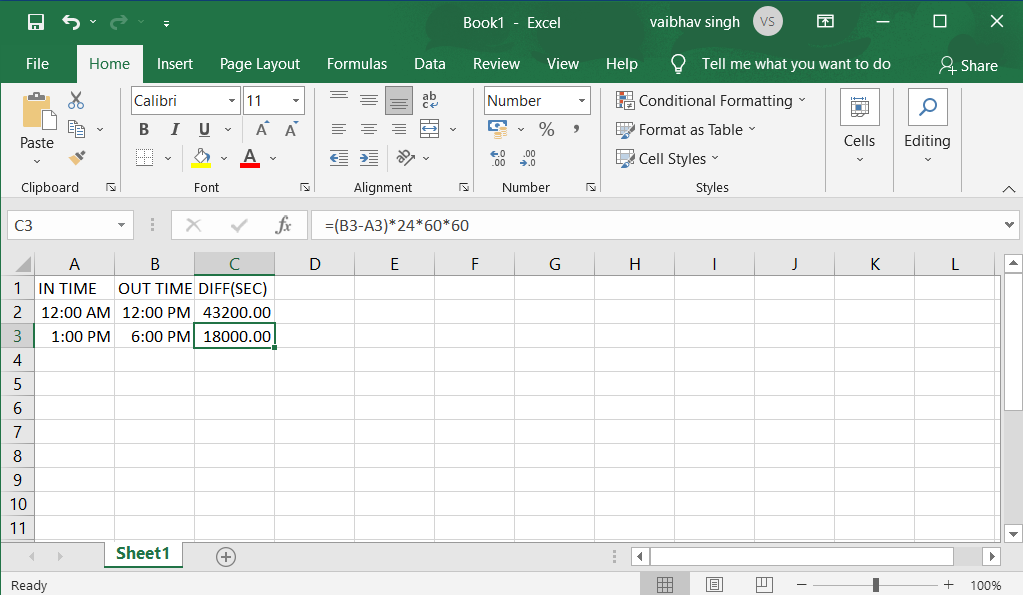### 3. Calculating Time Difference in Text Function:

Another easy way to quickly get the time difference without changing the format is to use the TEXT function.

`=TEXT(End Date - Start Date, Format)`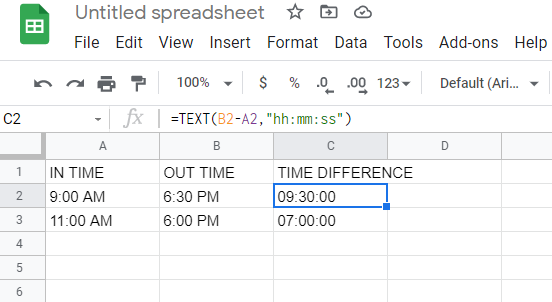### 4. Get the Time Difference in One-Unit (Hours/Minutes) and Ignore Others:

If you want to calculate the time difference between the two time-values in only the number of hours or minutes or seconds, then you can use the dedicated HOUR, MINUTE, or SECOND function.

Each of these functions takes one single argument, which is the time value and returns the specified time unit.

1. Calculating Hours Elapsed Between two times

`=HOUR(B3-A3)`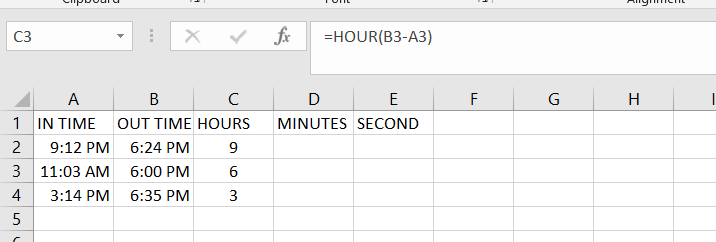2. Calculating Minutes from the time value result (excluding the completed hours):

Use the following formula to calculate Minutes from time value results excluding the completed hours:

`=MINUTE(B3-A3)`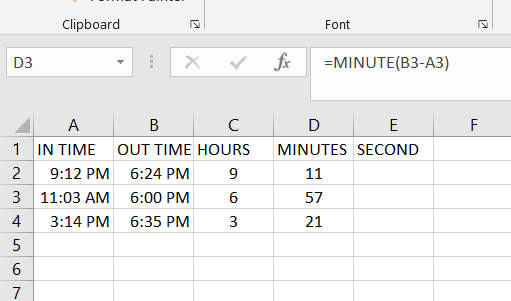3. Calculating Seconds from the time value result (excluding the completed hours and minutes):

Use the below formula to calculate Seconds from the time value result, excluding the completed hours and minutes:

`=SECOND(B4-A4)`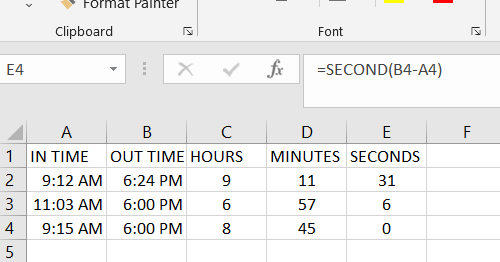### 5. Calculate elapsed time Till Now (from the start time):

If the user wants to calculate the total time that has elapsed between the start time and the current time, you can use the NOW formula instead of the End time.

NOW function returns the current date and the time in the cell in which it is used. It’s one of those functions that does not take any input argument.

`=NOW() - Start Time`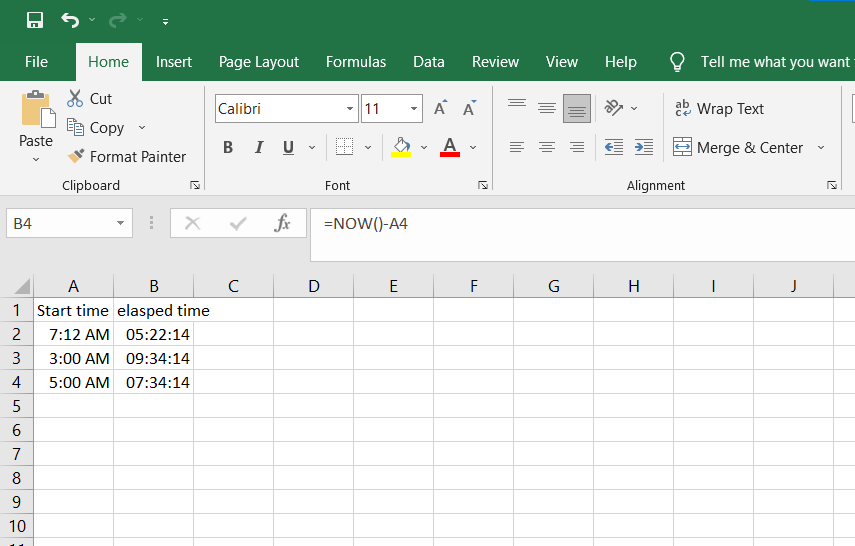### 6. Calculate and display negative times in Excel

Check if the time difference (A2-B2) is greater than 0, and if it is, they return that difference. If the time difference is less than zero, the first formula calculates the absolute difference.

`=IF(A2-B2>0, A2-B2, TEXT(ABS(A2-B2),"-h:mm"))`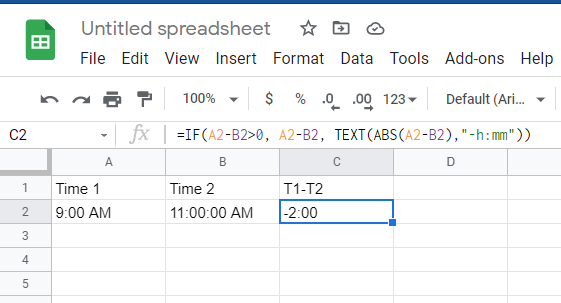Negative Date Value:

A date or time value cannot be negative in Excel. In case you are calculating the time difference and it turns out to be negative, Excel will show you hash symbols.

3. Adding and subtracting time in Excel: Using TIME function and using basic arithmetic

### 7. Adding and subtracting time in Excel: Using TIME function

The TIME function in Excel takes the hour value, the minute value, and the seconds value and converts it into a decimal number that represents this time.

For example, if the user wants to add 5 hours to an existing time,

`=Start Time + TIME(5,0,0)`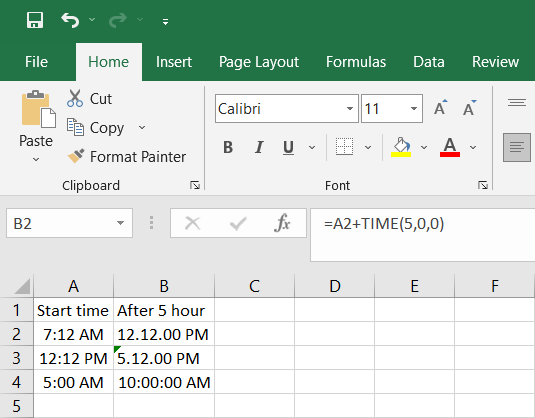• Sum of all time in Excel:

To add up all the time value in Excel we use the SUM function

`=SUM(A2:A6)`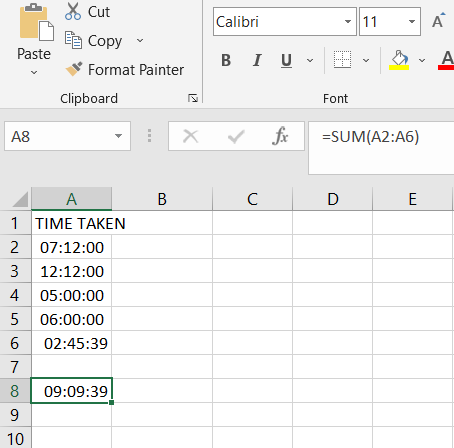### 8. Adding and subtracting time in Excel: Using Basic Athematic

When the time function is easy and convenient to use, it does come with a few restrictions (as covered above). The concept is simple – convert the time value into a decimal value that represents the portion of the day, and then you can add it to any time value in Excel.

For example, if you want to add 30 hours to an existing time value, you can use the below formula:

`=Start_time + 30/24`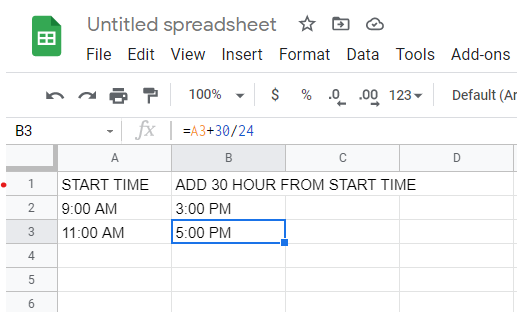Now taking the same concept forward let’s say you want to add 30 hours to a time value, you can use the below formula:

`=Start_time + (Number of hours to be added)/24`

The above formula does the same thing, where the integer part of the (30/24) would represent the total number of days in the time that you want to add, and the decimal part would represent the hours/minutes/seconds.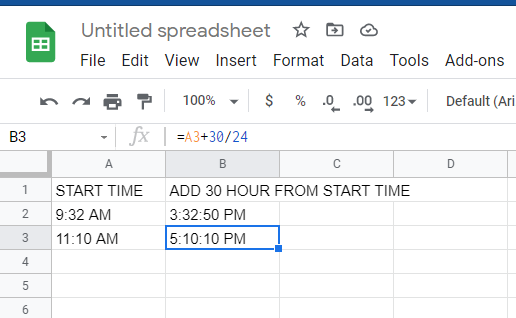Note: For, subtraction the same method is followed instead of + use – sign.

### 9. Summing over 24 hours in Excel:

Convert the time value into a decimal value that represents the portion of the day, and then you can add it to any time value in Excel.

For example, if you want to add 24 hours to an existing time value, you can use the below formula:

`=Start_time + 24/24`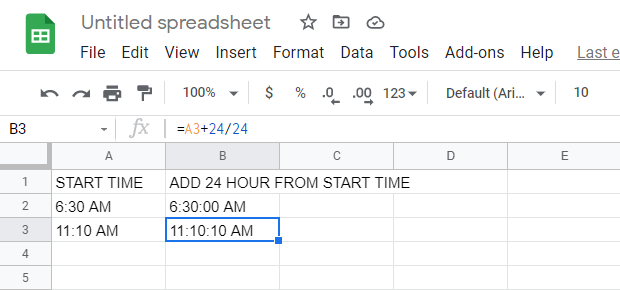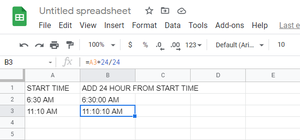Now taking the same concept forward let’s say you want to add as many hours to a time value, you can use the below formula:

`=Start_time + (Number of hour to be added)/24`

### 10. Calculate time when the date changes:

If there are not only hours, minutes and seconds but also dates in the columns, by selecting a blank cell, in this case, we select Cell C2, type this formula =B2-A2 (the time in Cell A2 is earlier than Cell B2, you can change them as you need).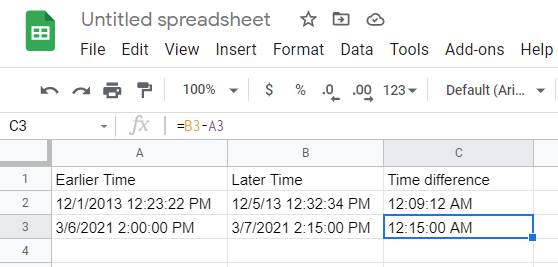## How excel handles date and time?

Regardless of how you have formatted a cell to display a date or time, Always Excel internally stores dates And times the same way. Excel stores dates and times as a number representing the number of days since 1900-Jan-0, plus a fractional portion of a 24 hour in the day in ddddd. tttttt format.

My Personal Notes arrow_drop_up